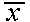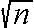The confidence interval around a sample mean gives confidence limits on the value of the population mean. This assumes that the sample mean was calculated from a random sample of the population and that the population is normally distributed. It is important to remember that ALL STATISTICS can have confidence intervals calculated around them, but the only one you may know about at this point in your statistical education is the confidence interval for the mean. Roughly speaking, a 95% confidence interval for the population mean can be calculated from a random sample by calculating the sample mean and sample standard deviation, using the standard deviation to calculate the (estimated) standard error of the sample mean, and then adding and subtracting two times the standard error to/from the sample mean. This will give you a reasonable estimate of the 95% confidence interval provided the sample is fairly large (say 30 or more). If the sample is smaller, the multiplier used will be greater than two. In a formula, it looks like this: confidence limits =± 2 * s /If the sample is genuinely random and from a normal distribution, after a 95% confidence interval is calculated around the sample mean, we can be 95% certain that the true mean (population mean) falls within the boundaries proscribed by the confidence interval (the confidence limits). If the sample is not random, however, then all bets are off! Return To Main Page